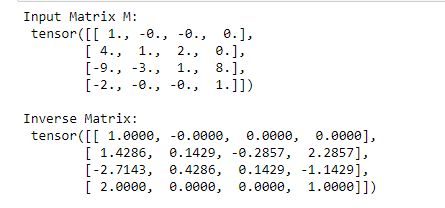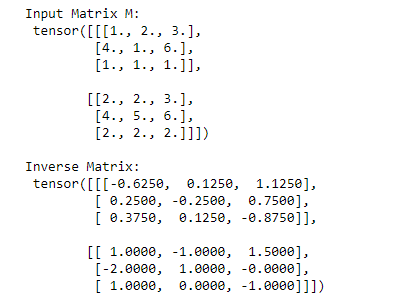# How to compute the inverse of a square matrix in PyTorch

• Last Updated : 13 Jun, 2022

In this article, we are going to cover how to compute the inverse of a square matrix in PyTorch

### torch.linalg.inv() method

we can compute the inverse of the matrix by using torch.linalg.inv() method. It accepts a square matrix and a batch of the square matrices as input. If the input is a batch of the square matrices then the output will also have the same batch dimensions. This method returns the inverse matrix.

Syntax: torch.linalg.inv(M)

Parameters:

• M – This is our square matrix or a batch of square matrix.

Returns: it will returns the inverse matrix.

### Example 1:

In this example, we will understand how to compute the inverse of a 4×4 square matrix in PyTorch.

## Python3

 `# import required library``import` `torch` `# define a 4x4 square matrix``mat ``=` `torch.tensor([[ ``1.00``, ``-``0.000``, ``-``0.00``,  ``0.00``],``        ``[ ``4.00``,  ``1.000``,  ``2.00``,  ``0.00``],``        ``[ ``-``9.00``,  ``-``3.00``,  ``1.00``,  ``8.00``],``        ``[ ``-``2.00``, ``-``0.00``, ``-``0.00``,  ``1.00``]])``print``(``"Input Matrix M: \n"``, mat)` `# compute the inverse of matrix``Mat_inv ``=` `torch.linalg.inv(mat)` `# display result``print``(``"\nInverse Matrix: \n"``, Mat_inv)`

Output:### Example 2:

In this example, we will compute the inverse of a batch of square matrices in PyTorch.

## Python3

 `# import required library``import` `torch` `# define a batch of two 3x3 square matrix``mat ``=` `torch.tensor([[[``1.0``, ``2.0``, ``3.0``], [``4.0``, ``1.0``, ``6.0``],``                     ``[``1.0``, ``1.0``, ``1.0``]],``                    ``[[``2.0``, ``2.0``, ``3.0``], [``4.0``, ``5.0``, ``6.0``],``                     ``[``2.0``, ``2.0``, ``2.0``]]])``print``(``"Input Matrix M: \n"``, mat)` `# compute the inverse of matrix``Mat_inv ``=` `torch.linalg.inv(mat)` `# display result``print``(``"\nInverse Matrix: \n"``, Mat_inv)`

Output:My Personal Notes arrow_drop_up